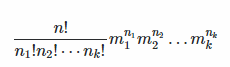Polyomino Combinatorics: How to count distinct n- letter long array permutations

Let’s say we have 3 arrays of following tetromino letters: J, L, O. Tetromino J is a 4 member long array (J,J,J,J), signifying the number of positions J block can take. L tetromino is also a 4 member long array and O is a square tetromino that has only one position (1 member long array). How do we calculate the total number of all unique n-letter long permutations, without programmatically permuting through them?

Example

Tetromino selection:

J, L, L, O, O

There is a number of unique permutations we can run through, such as:

J,L,L,O,O
or
L,L,O,J,O
...

Solution

The full answer to this problem is described by Y. Forman in the following math.stackoverflow post.

Essentially the formula looks like this, where the total number of possible strings is:So, given we have the following 5 tetromino blocks:
J,L,L,O,O – 5 letters

Each block has the following number of possible states:
J – 4
L – 4
L – 4
O – 1
O – 1

Now let’s group them:
1 x J and it has 4 states =
2 x L where each has 4 states =
2 x O where each has 1 state =

Calculation
(5! / (1! x 2! x 2!)) x (4¹ x 4² x 1²)
(120 / 4) x (4 x 16 x 1)
(30) x (64)
1920

There is a total number of 1,920 possible distinct tetromino combinations!

The above math was used in my JavaScript based Polyomino Block Puzzle Calculator.